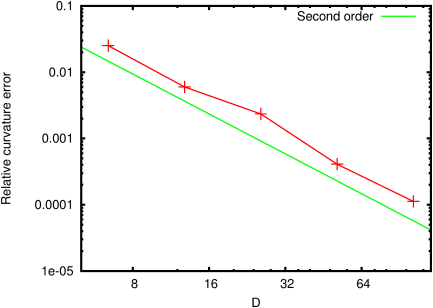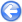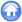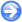### 9.1PASS: Circular droplet in equilibrium

Author
Stéphane Popinet
Command
sh spurious.sh spurious.gfs 4e-10
Version
1.1.2
Required files
spurious.sh convergence.ref kconvergence.ref
Running time
6 minutes 46 seconds

A circular droplet of diameter D=0.8 is initialised centered on the top-left corner of the unit box. Surface tension is imposed on the interface. The exact solution is given by Laplace’s law: uniform zero velocity and a pressure jump accross the interface exactly balancing the surface tension force.

The initial condition – while close to the exact solution – does not guarantee the exact balance between the numerical discretisations of surface tension and pressure gradient. However, these small initial perturbations generate small capillary waves which are progressively (on a timescale of order D2/µ) damped by viscosity so that the exact (to round-off error) balance is eventually obtained.

The convergence is obtained for a wide range of Laplace numbers La=σρ D2, as illustrated on Figure 106. Correspondingly, convergence of the curvature to a constant value is also obtained at all Laplace numbers as illustrated on Figure 107.

Figure 108 illustrates the convergence of the error on the droplet shape as a function of resolution for a Laplace number of 12000. Both the shape error and the relative error on the equilibrium curvature value illustrated on Figure 109 show close to second-order convergence.

 Figure 106: Evolution of the amplitude of the capillary currents max(|u|)(D/σ)1/2 as a function of non-dimensional time τ=tµ/D2 for the range of Laplace numbers indicated in the legend.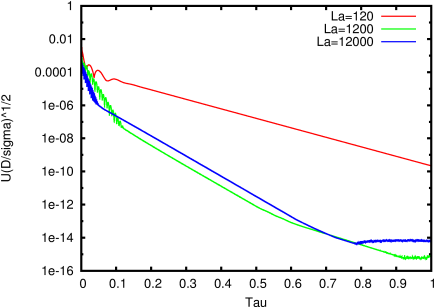Figure 107: Evolution of the standard deviation of the value of the curvature along the interface as a function of non-dimensional time τ=tµ/D2 for the range of Laplace numbers indicated in the legend.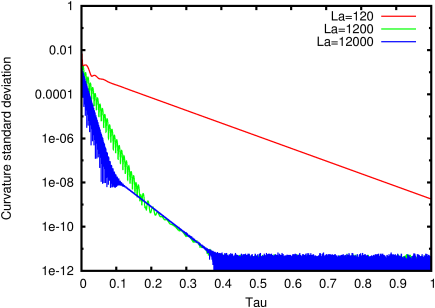Figure 108: Convergence of the error on the equilibrium shape of the droplet with resolution. The diameter is given in number of grid points.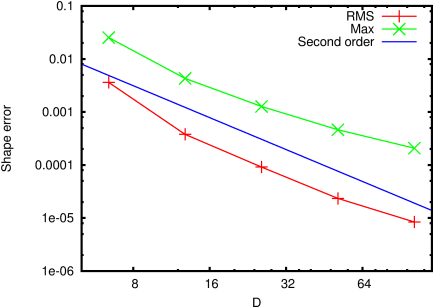Figure 109: Convergence of the relative error on the equilibrium curvature value with resolution. The diameter is given in number of grid points.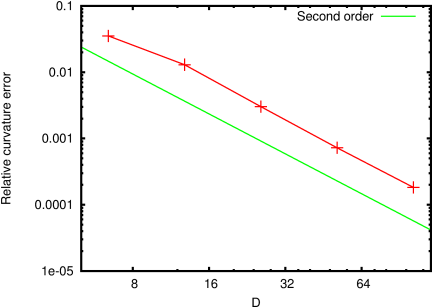#### 9.1.1PASS: Axisymmetric spherical droplet in equilibrium

Author
Stéphane Popinet
Command
sh ../spurious.sh axi.gfs 5e-8
Version
1.3.1
Required files
convergence.ref kconvergence.ref
Running time
17 minutes 22 seconds

The same test case but using the axisymmetric solver. The results are comparable.

 Figure 110: Evolution of the amplitude of the capillary currents max(|u|)(D/σ)1/2 as a function of non-dimensional time τ=tµ/D2 for the range of Laplace numbers indicated in the legend.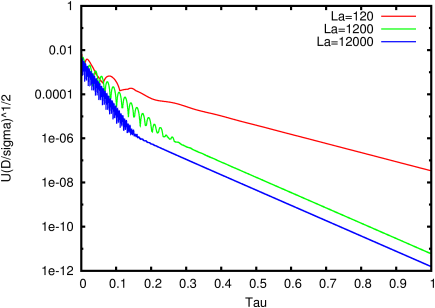Figure 111: Evolution of the standard deviation of the value of the curvature along the interface as a function of non-dimensional time τ=tµ/D2 for the range of Laplace numbers indicated in the legend.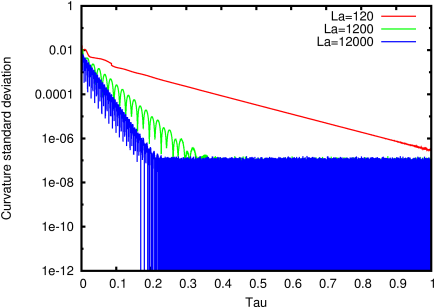Figure 112: Convergence of the error on the equilibrium shape of the droplet with resolution. The diameter is given in number of grid points.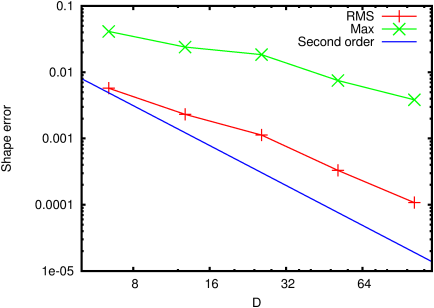Figure 113: Convergence of the relative error on the equilibrium curvature value with resolution. The diameter is given in number of grid points.